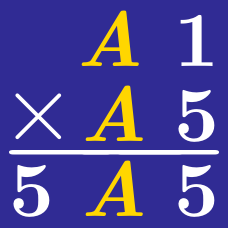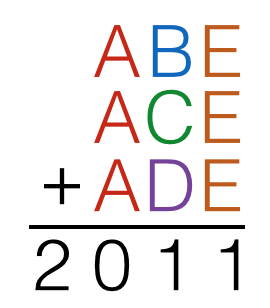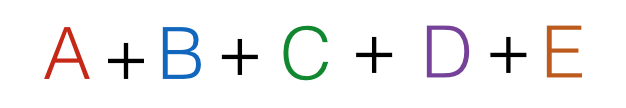Logic

# Cryptograms: Level 1 Challenges

$\Large \begin{array} {c c c } & \color{#3D99F6}A & \color{#D61F06}B & \color{#EC7300}C \\ \times& & & 8 \\ \hline \color{#3D99F6}A & \color{#EC7300}C & \color{#EC7300}C & \color{#EC7300}C \\ \end{array}$

In the cryptogram above, $A$, $B$, and $C$ are distinct digits. Find the value of $A+B+C+8.$

If $A, B, C,$ and $D$ are distinct digits, find the maximum possible value of the following sum:

$\begin{array} {ccc} & A & B \\ + & C & D \\ \hline \end{array}$

$\large{\begin{array}{lllllllll}&&&&&&&1&1&4&X&9\\\times&&&&&&&1&X&8&2&1\\\hline &\ &&1&2&3&4&5&6&7&8&9 \\\hline\end{array}}$

Above shows an incomplete long multiplication for which X represents a single digit integer. What is the value of X?Find$\begin{array} { l l l } & & & X & Y & Z \\ & & & X & Y & Z \\ &+ & & X & Y & Z \\ \hline & & & Z & Z & Z \\ \end{array}$

If $X, Y,$ and $Z$ are distinct digits, then what is the value of $X \times Y \times Z ?$

×